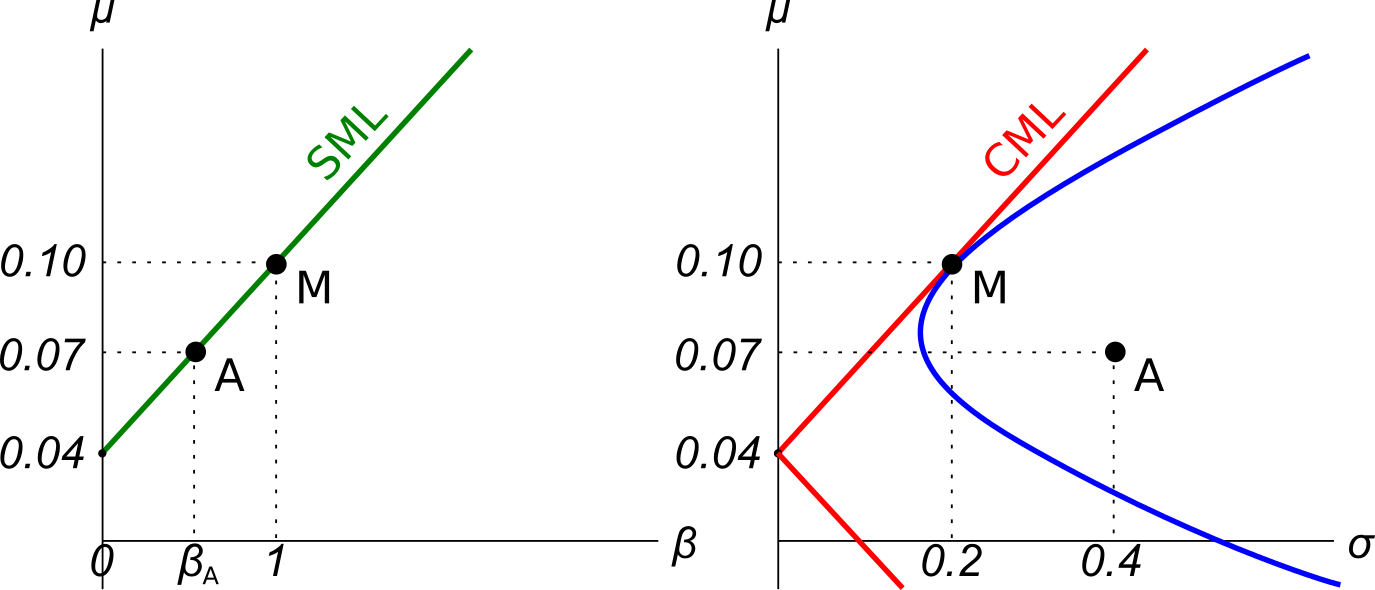# Fight Finance

#### CoursesTagsRandomAllRecentScores

All things remaining equal, according to the capital asset pricing model, if the systematic variance of an asset increases, its required return will increase and its price will decrease.
If the idiosyncratic variance of an asset increases, its price will be unchanged.

What is the relationship between the price of a call or put option and the total, systematic and idiosyncratic variance of the underlying asset that the option is based on? Select the most correct answer.

Call and put option prices increase when the:

A stock's required total return will increase when its:

A stock's required total return will decrease when its:

A stock's total standard deviation of returns is 20% pa. The market portfolio's total standard deviation of returns is 15% pa. The beta of the stock is 0.8.

What is the stock's diversifiable standard deviation?

Which of the following statements about returns is NOT correct? A stock's:

The capital market line (CML) is shown in the graph below. The total standard deviation is denoted by σ and the expected return is μ. Assume that markets are efficient so all assets are fairly priced.Which of the below statements is NOT correct?

A graph of assets’ expected returns $(\mu)$ versus standard deviations $(\sigma)$ is given in the graph below. The CML is the capital market line.Which of the following statements about this graph, Markowitz portfolio theory and the Capital Asset Pricing Model (CAPM) theory is NOT correct?

Examine the graphs below. Assume that asset A is a single stock. Which of the following statements is NOT correct? Asset A:The Capital Asset Pricing Model (CAPM) and the Single Index Model (SIM) are single factor models whose only risk factor is the market portfolio’s return. Say a Solar electricity generator company and a Beach bathing chair renting company are influenced by two factors, the market portfolio return and cloud cover in the sky. When it's sunny and not cloudy, both the Solar and Beach companies’ stock prices do well. When there’s dense cloud cover and no sun, both do poorly. Assume that cloud coverage risk is a systematic risk that cannot be diversified and that cloud cover has zero correlation with the market portfolio’s returns.

Which of the following statements about these two stocks is NOT correct?

The CAPM and SIM:

A common phrase heard in financial markets is that ‘high risk investments deserve high returns’. To make this statement consistent with the Capital Asset Pricing Model (CAPM), a high amount of what specific type of risk deserves a high return?

Investors deserve high returns when they buy assets with high: